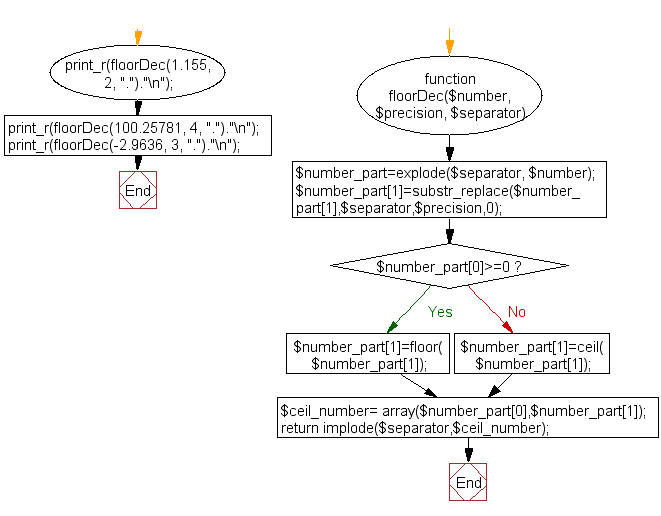﻿ PHP Array Exercise: Floor Decimal numbers with precision - w3resource# PHP Array Exercises : Floor decimal numbers with precision

## PHP Array: Exercise-18 with Solution

Write a PHP function to floor decimal numbers with precision.

Note: Accept three parameters number, precision, and \$separator
Sample Data :
1.155, 2, "."
100.25781, 4, "."
-2.9636, 3, "."

Sample Solution:

PHP Code:

``````<?php
function floorDec(\$number, \$precision, \$separator)
{
\$number_part=explode(\$separator, \$number);
\$number_part=substr_replace(\$number_part,\$separator,\$precision,0);
if(\$number_part>=0)
{\$number_part=floor(\$number_part);}
else
{\$number_part=ceil(\$number_part);}

\$ceil_number= array(\$number_part,\$number_part);
return implode(\$separator,\$ceil_number);
}
print_r(floorDec(1.155, 2, ".")."\n");
print_r(floorDec(100.25781, 4, ".")."\n");
print_r(floorDec(-2.9636, 3, ".")."\n");
?>
```
```

Sample Output:

```1.15
100.2578
-2.964
```

Flowchart:PHP Code Editor:

What is the difficulty level of this exercise?

﻿

## PHP: Tips of the Day

PHP: Anonymous recursive PHP functions

In order for it to work, you need to pass \$factorial as a reference

```\$factorial = function( \$n ) use ( &\$factorial ) {
if( \$n == 1 ) return 1;
return \$factorial( \$n - 1 ) * \$n;
};
print \$factorial( 5 );
```

Ref : https://bit.ly/38dj7jm